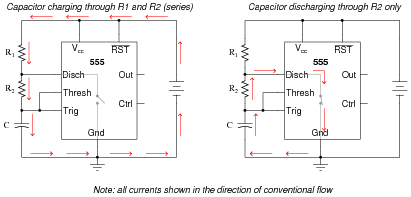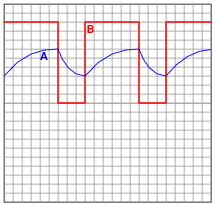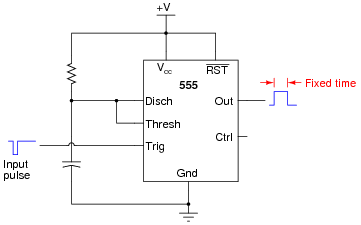# Timer Circuits

## Digital Circuits

• #### Question 1

 Don’t just sit there! Build something!!

Learning to analyze digital circuits requires much study and practice. Typically, students practice by working through lots of sample problems and checking their answers against those provided by the textbook or the instructor. While this is good, there is a much better way.

You will learn much more by actually building and analyzing real circuits, letting your test equipment provide the “answers” instead of a book or another person. For successful circuit-building exercises, follow these steps:

1. Draw the schematic diagram for the digital circuit to be analyzed.
2. Carefully build this circuit on a breadboard or other convenient medium.
3. Check the accuracy of the circuit’s construction, following each wire to each connection point, and verifying these elements one-by-one on the diagram.
4. Analyze the circuit, determining all output logic states for given input conditions.
5. Carefully measure those logic states, to verify the accuracy of your analysis.
6. If there are any errors, carefully check your circuit’s construction against the diagram, then carefully re-analyze the circuit and re-measure.

Always be sure that the power supply voltage levels are within specification for the logic circuits you plan to use. If TTL, the power supply must be a 5-volt regulated supply, adjusted to a value as close to 5.0 volts DC as possible.

One way you can save time and reduce the possibility of error is to begin with a very simple circuit and incrementally add components to increase its complexity after each analysis, rather than building a whole new circuit for each practice problem. Another time-saving technique is to re-use the same components in a variety of different circuit configurations. This way, you won’t have to measure any component’s value more than once.

• #### Question 2

The following schematic diagram shows a timer circuit made from a UJT and an SCR:Together, the combination of R1, C1, R2, R3, and Q1 form a relaxation oscillator, which outputs a square wave signal. Explain how a square wave oscillation is able to perform a simple time-delay for the load, where the load energizes a certain time after the toggle switch is closed. Also explain the purpose of the RC network formed by C2 and R4.

• #### Question 3

The model “555” integrated circuit is a very popular and useful “chip” used for timing purposes in electronic circuits. The basis for this circuit’s timing function is a resistor-capacitor (RC) network:In this configuration, the “555” chip acts as an oscillator: switching back and forth between “high” (full voltage) and “low” (no voltage) output states. The time duration of one of these states is set by the charging action of the capacitor, through both resistors (R1 and R2 in series). The other state’s time duration is set by the capacitor discharging through one resistor (R2):Obviously, the charging time constant must be τcharge = (R1 + R2)C, while the discharging time constant is τdischarge = R2C. In each of the states, the capacitor is either charging or discharging 50% of the way between its starting and final values (by virtue of how the 555 chip operates), so we know the expression e[(−t)/(τ)] = 0.5, or 50 percent.

Develop two equations for predicting the “charge” time and “discharge” time of this 555 timer circuit, so that anyone designing such a circuit for specific time delays will know what resistor and capacitor values to use.

### Footnotes:

For those who must know why, the 555 timer in this configuration is designed to keep the capacitor voltage cycling between 1/3 of the supply voltage and 2/3 of the supply voltage. So, when the capacitor is charging from 1/3VCC to its (final) value of full supply voltage (VCC), having this charge cycle interrupted at 2/3VCC by the 555 chip constitutes charging to the half-way point, since 2/3 of half-way between 1/3 and 1. When discharging, the capacitor starts at 2/3VCC and is interrupted at 1/3VCC, which again constitutes 50% of the way from where it started to where it was (ultimately) headed
.

• #### Question 4

The type “555” integrated circuit is a highly versatile timer, used in a wide variety of electronic circuits for time-delay and oscillator functions. The heart of the 555 timer is a pair of comparators and an S-R latch:The various inputs and outputs of this circuit are labeled in the above schematic as they often appear in datasheets (“Thresh” for threshold, “Ctrl” or “Cont” for control, etc.).

To use the 555 timer as an astable multivibrator, simply connect it to a capacitor, a pair of resistors, and a DC power source as such:If were were to measure the voltage waveforms at test points A and B with a dual-trace oscilloscope, we would see the following:Explain what is happening in this astable circuit when the output is “high,” and also when it is “low.”

• #### Question 5

This astable 555 circuit has a potentiometer allowing for variable duty cycle:With the diode in place, the output waveform’s duty cycle may be adjusted to less than 50% if desired. Explain why the diode is necessary for that capability. Also, identify which way the potentiometer wiper must be moved to decrease the duty cycle.

• #### Question 6

A popular use of the 555 timer is as a monostable multivibrator. In this mode, the 555 will output a pulse of fixed length when commanded by an input pulse:How low does the triggering voltage have to go in order to initiate the output pulse? Also, write an equation specifying the width of this pulse, in seconds, given values of R and C. Hint: the magnitude of the supply voltage is irrelevant, so long as it does not vary during the capacitor’s charging cycle. Show your work in obtaining the equation, based on equations of RC time constants. Don’t just copy the equation from a book or datasheet!

• #### Question 7

A sequential timer circuit may be constructed from multiple 555 timer ICs cascaded together. Examine this circuit and determine how it works:Can you think of any practical applications for a circuit such as this?

• #### Question 8

Predict how the operation of this astable 555 timer circuit will be affected as a result of the following faults. Specifically, identify what will happen to the capacitor voltage (VC1) and the output voltage (Vout) for each fault condition. Consider each fault independently (i.e. one at a time, no multiple faults):Resistor R1 fails open:
Solder bridge (short) across resistor R1:
Resistor R2 fails open:
•Solder bridge (short) across resistor R2:
Capacitor C1 fails shorted:

For each of these conditions, explain why the resulting effects will occur.

• #### Question 9

This circuit uses a “555” integrated circuit to produce a low-frequency square-wave voltage signal (seen between the “Out” terminal of the chip and ground), which is used to turn a pair of transistors on and off to flash a large lamp. Predict how this circuit will be affected as a result of the following faults. Consider each fault independently (i.e. one at a time, no multiple faults):Transistor Q1 fails open (collector-to-emitter):
Transistor Q2 fails open (collector-to-emitter):
Resistor R3 fails open:
Transistor Q1 fails shorted (collector-to-emitter):

For each of these conditions, explain why the resulting effects will occur.

• #### Question 10

What would happen to the operation of this astable 555 timer circuit if a resistor were accidently connected between the “Control” terminal and ground? Explain the reason for your answer.• #### Question 11

A student builds their first astable 555 timer circuit, using a TLC555CP chip. Unfortunately, it seems to have a problem. Sometimes, the output of the timer simply stops oscillating, with no apparent cause. Stranger yet, the problem often occurs at the precise time anyone moves their hand within a few inches of the circuit board (without actually touching anything!).

What could the student have done wrong in assembling this circuit to cause such a problem? What steps would you take to troubleshoot this problem?

• #### Question 12

Identify at least one component fault that would cause the final 555 timer output to always remain low:For each of your proposed faults, explain why it will cause the described problem.

• #### Question 13

Pulse Width Modulation, or PWM, is a very popular means of controlling power to an electrical load such as a light bulb or a DC motor. With PWM control, the duty cycle of a high-frequency digital (on/off) signal is varied, with the effect of varying power dissipation at the load:One of the major advantages to using PWM to proportion power to a load is that the final switching transistor operates with minimal heat dissipation. If we were to use a transistor in its linear (“active”) mode, it would dissipate far more heat when controlling the speed of this motor! By dissipating less heat, the circuit wastes less power.

Explain why the power transistor in this circuit runs cooler when buffering the PWM signal from the 555 timer, rather than if it were operated in linear mode. Also, identify which direction the potentiometer wiper must be moved to increase the speed of the motor.

Challenge question: suppose we needed to control the power of a DC motor, when the motor’s operating voltage was far in excess of the 555 timer’s operating voltage. Obviously, we need a separate power supply for the motor, but how would we safely interface the 555’s output with the power transistor to control the motor speed? Draw a schematic diagram to accompany your answer.

• #### Question 14

It is common to see a capacitor connected between the “Control” terminal and ground in 555 timer circuits, especially when precise timing is important.Explain what purpose the capacitor C2 serves in this circuit.

• #### Question 15

Special integrated circuits called delay elements or delay gates are manufactured to provide nanoseconds’ worth of intentional time delays in digital circuits. Identify a part number for such an IC, research its datasheet, and describe an application where one might be needed.

• #### Question 16

An important measurement of pulse waveforms is duty cycle. Give a precise. mathematical definition for this term.

Also, write an equation solving for pulse width given duty cycle (D) and frequency (f).

• #### Question 17

Research the “pinout” for a 555 timer integrated circuit, in an 8-pin DIP package. Then, label the pins as shown on this figure: